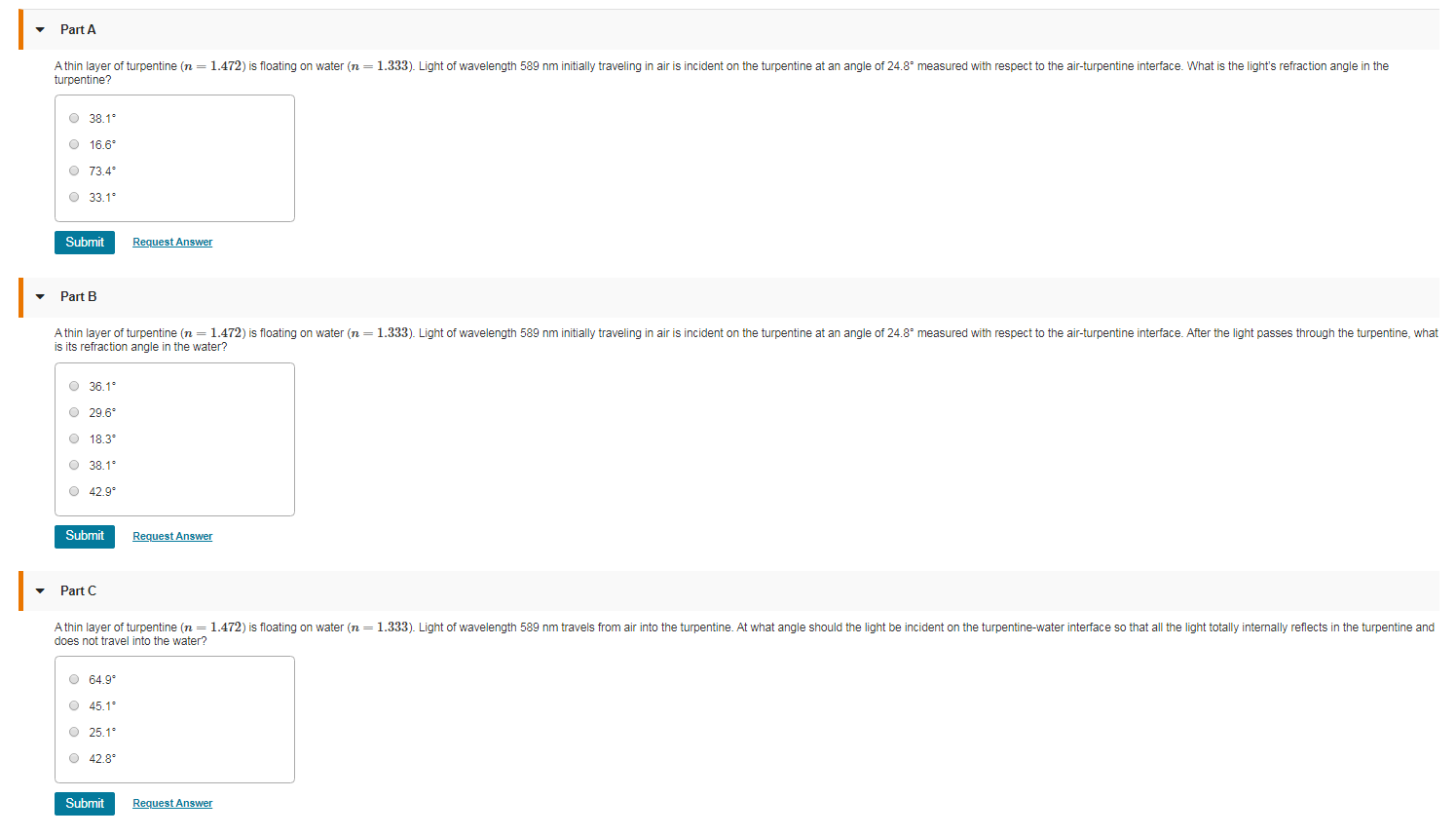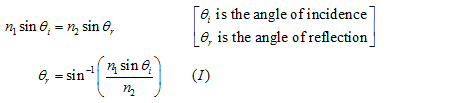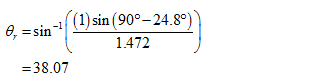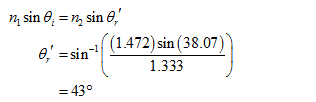# Part AA thin layer of turpentine (n = 1.472) is floating on water (n = 1.333). Light of wavelength 589 nm initially traveling in air is incident on the turpentine at an angle of 24.8° measured with respect to the air-turpentine interface. What is the light's refraction angle in theturpentine?O 38.1°O 16.6°O 73.4*O 33.1"SubmitRequest AnswerPart BA thin layer of turpentine (n = 1.472) is floating on water (n = 1.333). Light of wavelength 589 nm initially traveling in air is incident on the turpentine at an angle of 24.8° measured with respect to the air-turpentine interface. After the light passes through the turpentine, whatis its refraction angle in the water?O 36.1°O 29.6°O 18.3O 38.1°O 42.9°SubmitRequest AnswerPart CA thin layer of turpentine (n = 1.472) is floating on water (n = 1.333). Light of wavelength 589 nm travels from air into the turpentine. At what angle should the light be incident on the turpentine-water interface so that all the light totally internally reflects in the turpentine anddoes not travel into the water?O 64.9°O 45.1°O 25.1°O 42.8°SubmitRequest Answer

Question
8 viewshelp_outlineImage TranscriptionclosePart A A thin layer of turpentine (n = 1.472) is floating on water (n = 1.333). Light of wavelength 589 nm initially traveling in air is incident on the turpentine at an angle of 24.8° measured with respect to the air-turpentine interface. What is the light's refraction angle in the turpentine? O 38.1° O 16.6° O 73.4* O 33.1" Submit Request Answer Part B A thin layer of turpentine (n = 1.472) is floating on water (n = 1.333). Light of wavelength 589 nm initially traveling in air is incident on the turpentine at an angle of 24.8° measured with respect to the air-turpentine interface. After the light passes through the turpentine, what is its refraction angle in the water? O 36.1° O 29.6° O 18.3 O 38.1° O 42.9° Submit Request Answer Part C A thin layer of turpentine (n = 1.472) is floating on water (n = 1.333). Light of wavelength 589 nm travels from air into the turpentine. At what angle should the light be incident on the turpentine-water interface so that all the light totally internally reflects in the turpentine and does not travel into the water? O 64.9° O 45.1° O 25.1° O 42.8° Submit Request Answer fullscreen
check_circle

Step 1

(a)

Apply  to snell’s  law at A.Substitute the values in equation (I).Step 2

(b)

Apply  to snell’s  law at B....

### Want to see the full answer?

See Solution

#### Want to see this answer and more?

Solutions are written by subject experts who are available 24/7. Questions are typically answered within 1 hour.*

See Solution
*Response times may vary by subject and question.
Tagged in